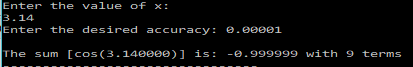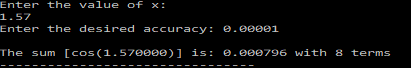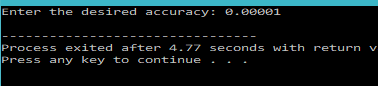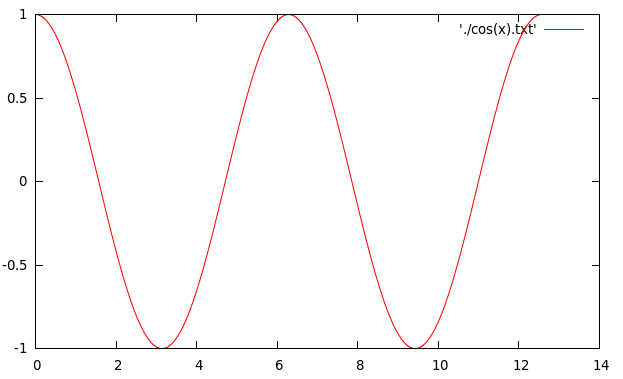# Evaluate and plot the Cosine (Infinite) Series using C Programming and Gnuplot

In the last post I discussed and showed you how to write a program that finds the sum of the Sine series(Infinite so to speak).

Using the same concept, we will extend it a bit further in this post.

In this post we will evaluate the Cosine series, correct upto a certain decimal places, for a given range of x in radians. We would store the value of Cos(x) evaluated in a text file and then plot them using Gnuplot.

So let’s first start with writing a program that evaluates the Cosine series.
The series is given by:$\cos (x) = 1 - \frac{x^2}{2!} + \frac{x^4}{4!} - ....$

Ratio of the successive terms(index the numbers from 0):$R=\frac{t_i}{t_{i-1}}= -\frac{x^2}{2i(2i-1)}$

Since, we indexed the terms starting from 0, therefore, for the above relation to work,$i$ will go from 1 to$n$.
Now, knowing the first($t_0$) term, the successive terms can be calculated as :$t_1=R\times t_0$$t_2=R\times t_1$

and so on.

Therefore, the C program that calculates the sum of the cosine series upto a certain accuracy can be written as shown below.

### PROGRAM:

/********************************
******INFINITE SERIES SUM**********
Series: cos(x) = 1 - (x^2/2!) + (x^4/4!) - .....
********************************/
#include<stdio.h>
#include<math.h>
main(){
int i=1;
double x,t0,t1,R,sum,eps;
printf("Enter the value of x:\n");
scanf("%lf",&x);
printf("Enter the desired accuracy: ");
scanf("%lf",&eps);
//Initialize First Term
t0=1;
//Make sum equal to the first term
sum=t0;
do{
//Find the ratio of the second term to the first term using already known relation
R=-(x*x)/(2*i-1)/(2*i);
//Calculate the second term
t1=R*t0;
//find the new sum
sum=sum+t1;
t0=t1;
i++;
//keep on summing terms until the required accuracy is reached
}while(fabs(t1/sum)>eps);
printf("\nThe sum [cos(%lf)] is: %lf with %d terms",x,sum,i);
}


### OUTPUT:

The program asks the user to enter the value of x and the desired accuracy, and gives answer.Now that we have a program for evaluating the cosine series, we can write a program that will evaluate the cosine series in a given range[0 to 4pi] and store the values in a file.

### PROGRAM:

/********************************
******INFINITE SERIES SUM**********
Series: cos(x) = 1 - (x^2/2!) + (x^4/4!) - .....
********************************/
#include<stdio.h>
#include<math.h>
main(){
FILE *fp=NULL;
fp=fopen("cos(x).txt","w");
double x,t0,t1,R,sum,eps;
printf("Enter the desired accuracy: ");
scanf("%lf",&eps);
for(x=0.0000;x<=4*M_PI;x=x+0.001){
int i=1;
//Initialize First Term
t0=1;
//Make sum equal to the first term
sum=t0;
do{
//Find the ratio of the second term to the first term using already known relation
R=-(x*x)/(2*i-1)/(2*i);
//Calculate the second term
t1=R*t0;
//find the new sum
sum=sum+t1;
t0=t1;
i++;
//keep on summing terms until the required accuracy is reached
}while(fabs(t1/sum)>eps);
fprintf(fp,"%lf\t%lf\n",x,sum);
}
}


### OUPTUT:

When you run the above C program it will ask for the accuracy desired for the calculations.
When the execution is complete, it will create a txt file called ‘cos(x).txt’ that will contain the data to be plotted.### Gnuplot Command:

You can plot the data using Gnuplot, by giving the following command:
plot './cos(x).txt' w l

### Gnuplot OUPTUT:[wpedon id="7041" align="center"]